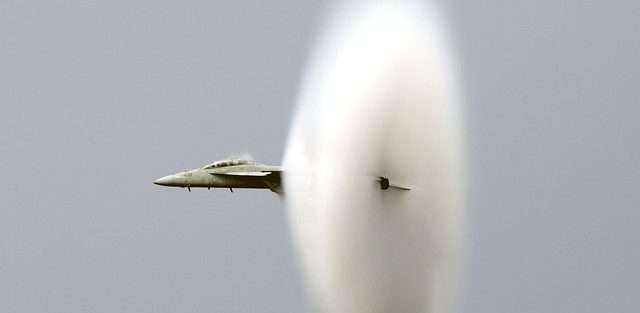# Mach numberThe Mach number is the ratio of the speed of an object to the speed of sound.

In aviation, the mach number is the ratio of the true air speed of the aircraft to local speed of sound, which can vary due to atmospheric conditions, air temperature and density. Mach speed is often used with a numeral, i.e. Mach 1, Mach 2.

If the aircraft is flying at Mach speed, it means it is flying equal to the speed of sound.  Like most ratios, there are approximations and categories depending on the speed of the object.

## How is the Mach number measured

The Mach number is measured with the use of a flight instrument known as a machmeter. Found in the cockpit of most high-speed aircraft, including private jets, a machmeter can be combined with an air speed indicator (ASI) as part of the jet’s air data computer.

Speed ratios are normally separated into the categories of subsonic, transonic, supersonic, and hypersonic, which classify air and spacecraft with different Mach numbers. A supersonic jet is able to speed faster than the speed of sound, therefore it is given the Mach number 1.

The world’s fastest private jet currently in production is the Cessna Citation X, which flies at a speed of 617 mph, or Mach speed of 0.92, just under the speed of sound.